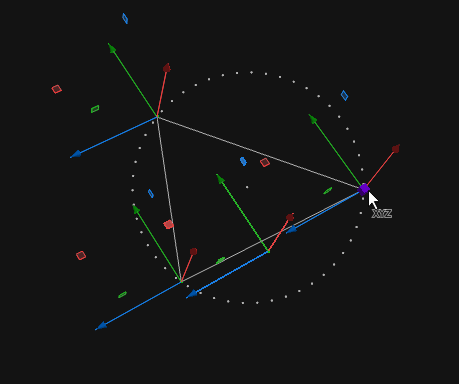## Recommended Posts

Hey everyone,

I am in the process of creating a digital asset and its basic building block is a circle. First I have the user plot three points in 3D space. And from these 3 points which form a triangle, I am creating a circle that circumscribes this triangle.

The algorithm I have so far from gathered resources are below;

1.) calculate the normal vector to the triangle formed by the three user-defined points.

2.) use a reference plane into which this triangle will rotate onto, say the XY plane

3.) rotate the three points into this reference plane after calculating and building a rotation matrix

4.) find the center of the circumscribing circle from the three points

5.) calculate the radius of the circle which is easy

6.) build the circle on the reference plane

7.) build a reverse rotation matrix to rotate the points back to the original plane

I'm not sure what I am doing wrong but the code I have so far works only if the points lie on a plane in 2D space. The minute the points have values in the 3'rd dimension, it gets wonky. I'm also not sure if the rotation matrix in Houdini does the rotation about the origin or about the pivot of the three points. I can share the code to take a look at it.

If anyone has any solutions or suggestions, kindly let me know! Much appreciated!

##### Share on other sites

sry here is the code

```vector CalcCircleCenter(vector pt1, pt2, pt3, center) {
float yDelta_a = pt2.y - pt1.y;
float xDelta_a = pt2.x - pt1.x;
float yDelta_b = pt3.y - pt2.y;
float xDelta_b = pt3.x - pt2.x;

if(abs(xDelta_a) <= 0.000000001 && abs(yDelta_b) <= 0.000000001) {
center.x = 0.5 * (pt2.x + pt3.x);
center.y = 0.5 * (pt1.y + pt2.y);
center.z = pt1.z;
return 1;
}

//the IsPerpendicular() assures that xDelta(s) are not zero
float aSlope = yDelta_a / xDelta_a;
float bSlope = yDelta_b / xDelta_b;

//checking whether the given points are colinear
if(abs(aSlope - bSlope) <= 0.000000001) {
return -1;
}

//calculate the center
center.x = (aSlope * bSlope * (pt1.y - pt3.y) + bSlope * (pt1.x + pt2.x) - aSlope * (pt2.x + pt3.x)) / (2 * (bSlope - aSlope));
center.y = -1 * (center.x - (pt1.x + pt2.x) / 2) / aSlope + (pt1.y + pt2.y) / 2;

return center;
}

//check if the given point is perpendicular to x or y axis
int IsPerpendicular(vector pt1, pt2, pt3) {
float yDelta_a= pt2.y - pt1.y;
float xDelta_a= pt2.x - pt1.x;
float yDelta_b= pt3.y - pt2.y;
float xDelta_b= pt3.x - pt2.x;

//check whether the line of the two pts are vertical
if (abs(xDelta_a) <= 0.000000001 && abs(yDelta_b) <= 0.000000001){
return false;
}

if (abs(yDelta_a) <= 0.000000001){
return true;
}
else if (abs(yDelta_b) <= 0.000000001){
return true;
}
else if (abs(xDelta_a) <= 0.000000001){
return true;
}
else if (abs(xDelta_b) <= 0.000000001){
return true;
}
else
return false ;
}

//find circle center based on a check
void FindCircleCenter(vector pt1, pt2, pt3, center) {
if(!IsPerpendicular(pt1, pt2, pt3)) {
CalcCircleCenter(pt1, pt2, pt3, center);
}
else if(!IsPerpendicular(pt1, pt3, pt2)) {
CalcCircleCenter(pt1, pt3, pt2, center);
}
else if(!IsPerpendicular(pt2, pt1, pt3)) {
CalcCircleCenter(pt2, pt1, pt3, center);
}
else if(!IsPerpendicular(pt2, pt3, pt1)) {
CalcCircleCenter(pt2, pt3, pt1, center);
}
else if(!IsPerpendicular(pt3, pt2, pt1)) {
CalcCircleCenter(pt3, pt2, pt1, center);
}
else if(!IsPerpendicular(pt3, pt1, pt2)) {
CalcCircleCenter(pt3, pt1, pt2, center);
}
else {
removepoint(0,0);
removepoint(0,1);
removepoint(0,2);
return;
}

removepoint(0,0);
removepoint(0,1);
removepoint(0,2);
}

//get the position of the three points circumscribing the triangle
vector A = point(@OpInput1,"P",0);
vector B = point(@OpInput1,"P",1);
vector C = point(@OpInput1,"P",2);

//initializing center of circumscribing circle
vector center = {0,0,0};

//calculating normal vector to the triangle formed by the 3 points
vector Delta_a = A - B;
vector Delta_b = C - B;

vector circle_normal = cross(normalize(Delta_b), normalize(Delta_a));
vector xy_normal = {0,0,1};

//rotation axis around which the circle will rotate into XY plane
vector rot_axis = cross(normalize(xy_normal),normalize(circle_normal));
float rot_angle = acos(dot(normalize(xy_normal),normalize(circle_normal))); //in radians

//building quaternion rotation matrix
float angle = cos(rot_angle * 0.5);
rot_axis *= sin(rot_angle * 0.5);

matrix3 rot_quat = ident();
rotate(rot_quat, angle, normalize(rot_axis));
quaternion(rot_quat);

//rotate points A, B and C
A *= rot_quat;
B *= rot_quat;
C *= rot_quat;
center *= rot_quat;

FindCircleCenter(A, B, C, center);

v@offset = center;

float deg_to_rad = 3.14159 / 180.0;

//making a primitive for the circle

//building reverse quaternion rotation
angle = cos(rot_angle * 0.5);

vector rot_back_axis = cross(normalize(xy_normal),normalize(circle_normal));
rot_back_axis *= sin(rot_angle * 0.5);

matrix3 rot_back_quat = ident();
rotate(rot_back_quat, angle, normalize(rot_back_axis));
quaternion(rot_back_quat);

//creating the circle
for(int i = 0; i<360; i++) {
center.z = 0;

//rotate the point back to original plane
center *= rot_back_quat;

}```

##### Share on other sites
```// Detail wrangle.

#define PI 3.1415926535897932384

vector a = point(0, "P", 0);
vector b = point(0, "P", 1);
vector c = point(0, "P", 2);

vector ac = c - a;
vector ab = b - a;
vector ab_x_ac = cross(ab, ac);
vector a_center = (cross(ab_x_ac, ab) * length2(ac) + cross(ac, ab_x_ac) * length2(ab)) / (2.0 * length2(ab_x_ac));

vector normal = normalize(ab_x_ac);
vector center = a + a_center;

// Test.
vector x, y, z=normal;
makebasis(x, y, z);
matrix3 r = set(x, y, z);

int count = 50;
for (int i = 0; i < count; i++)
{
float angle = 2 * PI / count * i;
vector pos = set(sin(angle), cos(angle), 0) * radius;
addpoint(0, pos * r + center);
}Here is working stuff found there. Add degeneracy testing if you need it.

circumcircle.hipnc

•7

### @f1480187

you sir are a JEDI!!!! Thanks so much!!!

Kind regards,
Naveen
SideFX Intern

## Create an account

Register a new account